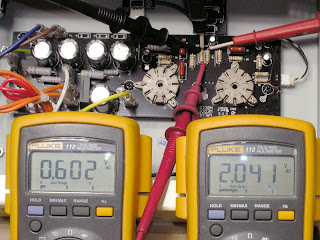## Friday, February 6, 2009

### Epiphone Valve Junior - Gain Reduction due to R6 and R7 in a Stock VJ

This post explains a particular part of the Epiphone Valve Junior circuit. If you're looking to reduce the volume and/or gain in your VJ, I started a series of posts for a gain reduction mod.

Here's a schematic for the stock Epiphone Valve Junior:There is a voltage divider in the preamp to reduce the overall gain of the amplifier. R6 and R7 are 1 Meg resistors used to form the circuit:These two resistors are in series with the output feeding the next stage being taken at their junction. This a straight ahead voltage divider circuit. R6 and R7 are indicated in red on the schematic below Click on it to see it in full detail.If you follow the math in the voltage divider posts, you'll see that with two resistors of equal value, as R6 and R7 are here, the voltage at the output will be 50% of the voltage at the input.

That would mean that with 2 Volts output from the first gain stage (V1) the voltage seen by the second stage (V2) would be just 1 Volt.

Things aren't quite that simple in the Valve Junior circuit though. If you look at the schematic you'll see that the 1 Meg volume pot is connected in parallel with R7:So the voltage divider is really composed of three resistors - R6 in series with the total resistance of R7 and the volume pot in parallel.

Ok, so that's a bit more complicated. We really want just two resistances to calculate the voltage divider output. Fortunately two resistors in parallel can be treated as a single resistance. We can find the effective resistance of R7 and VR1 in parallel using the following formula:If this formula seems too daunting, the Champion 600 Fat Boost Mod Resistor Values post goes though the details of how to apply it.

Using this formula you'll find that any two resistors of equal value connected in parallel will have a combined value of one half the value of a single resistor.

Incidentally, this is what guides the rule of thumb about connecting speakers in parallel (e.g. two 8 ohm speakers connected in parallel yields a 4 ohm load).

Here we're luck and the two are equal with R7 being 1 Meg and the end to end resistance of the volume pot being 1 Meg. One Megaohm is one million ohms. Half of one million is 500,000 ohms. One thousand ohms is one Kilohm, so the effective resistance of the two in parallel is 500 Kilohms or 500K.

Here is a modified schematic with the parallel resistance of R7 and VR1 shown a a single 500K component:As far as the Valve Junior's functioning is concerned this simplified circuit is the same as the original circuit even though it doesn't indicate the actual physical components. This is what's called an equivalent circuit, and we use it to make the functioning of the circuit easier to comprehend.

So after all of that we have new values for the voltage divider. The top half is still the value of R6 - 1 Meg. The bottom half is now the equivalent resistance of R7 and VR1 in parallel, or 500k.

Using a form of the voltage divider formula we'll find that the output voltage will be about 30% of the input voltage. So with the 2 Volts input given in the example above, our output voltage should be 2 Volts times .3 which equals .6 volts.

You may notice that this .6 Volts is itself the input to another voltage divider - the volume pot itself. You can see how a pot acts a voltage divider in part 3 of the voltage divider post.

So after all that math, it's time for a reality check. Here the right hand meter is connected from the bottom of R7 to the top of R6 - effectively measuring the input voltage to the divider. The left hand meter is connected across R7 - effectively measuring the output voltage feeding the volume pot:With enough signal applied to achieve 2 Volts from stage 1, the output voltage feeding stage 2 is .6 Volts - right in line with our calculations.

That's a gain reduction in the stock Valve Junior of about 10 dB. That means, of course, that eliminating the voltage divider by jumpering over R6 will result in a 10 dB increase in gain.

So now you know what that 1 Meg R6 is doing in your Valve Junior. Whether or not you want to keep it there is another story entirely.